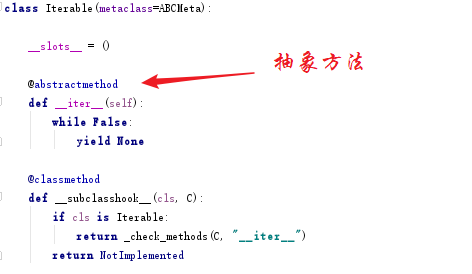# 2.7 从生成器使用入门协程¶## 1. 可迭代、迭代器、生成器¶

import collections
from collections.abc import Iterable, Iterator, Generator

# 字符串
astr = "XiaoMing"
print("字符串：{}".format(astr))
print(isinstance(astr, Iterable))
print(isinstance(astr, Iterator))
print(isinstance(astr, Generator))

# 列表
alist = [21, 23, 32,19]
print("列表：{}".format(alist))
print(isinstance(alist, Iterable))
print(isinstance(alist, Iterator))
print(isinstance(alist, Generator))

# 字典
adict = {"name": "小明", "gender": "男", "age": 18}

# deque


字符串：XiaoMing
True
False
False

True
False
False

True
False
False

deque：deque(['a', 'b', 'c', 'd', 'e', 'f', 'g'])
True
False
False


1. 可以通过，dir()方法查看，若有有__iter__说明是可迭代的，但是如果没有，也不能说明不可迭代，原因见第二条。

2. 判断是否可迭代，不能仅看是否有__iter__ 来草率决定，因为只实现了__getitem__ 方法的也有可能是可迭代的。因为当没有__iter__时， Python 解释器会去找__getitem__，尝试按顺序（从索引0开始）获取元素，不抛异常，即是可迭代。

3. 所以，最好的判断方法应该是通过 for循环或者iter() 去真实运行。from collections.abc import Iterable, Iterator, Generator

class MyList(object):  # 定义可迭代对象类

def __init__(self, num):
self.end = num  # 上边界

# 返回一个实现了__iter__和__next__的迭代器类的实例
def __iter__(self):
return MyListIterator(self.end)

class MyListIterator(object):  # 定义迭代器类

def __init__(self, end):
self.data = end  # 上边界
self.start = 0

# 返回该对象的迭代器类的实例；因为自己就是迭代器，所以返回self
def __iter__(self):
return self

# 迭代器类必须实现的方法，若是Python2则是next()函数
def __next__(self):
while self.start < self.data:
self.start += 1
return self.start - 1
raise StopIteration

if __name__ == '__main__':
my_list = MyList(5)  # 得到一个可迭代对象
print(isinstance(my_list, Iterable))  # True
print(isinstance(my_list, Iterator))  # False
# 迭代
for i in my_list:
print(i)

my_iterator = iter(my_list)  # 得到一个迭代器
print(isinstance(my_iterator, Iterable))  # True
print(isinstance(my_iterator, Iterator))  # True

# 迭代
print(next(my_iterator))
print(next(my_iterator))
print(next(my_iterator))
print(next(my_iterator))
print(next(my_iterator))


0
1
2
3
4

True
False

True
True

0
1
2
3
4


from collections.abc import Iterator

aStr = 'abcd'  # 创建字符串，它是可迭代对象
aIterator = iter(aStr)  # 通过iter()，将可迭代对象转换为一个迭代器
print(isinstance(aIterator, Iterator))  # True
next(aIterator)  # a
next(aIterator)  # b
next(aIterator)  # c
next(aIterator)  # d


1. 迭代器，是其内部实现了，__next__ 这个魔术方法。(Python3.x)

2. 可以通过，dir()方法来查看是否有__next__来判断一个变量是否是迭代器的。

yield 是什么东西呢，它相当于我们函数里的return。在每次next()，或者for遍历的时候，都会yield这里将新的值返回回去，并在这里阻塞，等待下一次的调用。正是由于这个机制，才使用生成器在Python编程中大放异彩。实现节省内存，实现异步编程。

# 使用列表生成式，注意不是[]，而是()
L = (x * x for x in range(10))
print(isinstance(L, Generator))  # True

• 实现yield的函数

# 实现了yield的函数
def mygen(n):
now = 0
while now < n:
yield now
now += 1

if __name__ == '__main__':
gen = mygen(10)
print(isinstance(gen, Generator))  # True


## 2. 如何运行/激活生成器¶

def mygen(n):
now = 0
while now < n:
yield now
now += 1

if __name__ == '__main__':
gen = mygen(4)

# 通过交替执行，来说明这两种方法是等价的。
print(gen.send(None))
print(next(gen))
print(gen.send(None))
print(next(gen))


0
1
2
3


## 3. 生成器的执行状态¶

from inspect import getgeneratorstate

def mygen(n):
now = 0
while now < n:
yield now
now += 1

if __name__ == '__main__':
gen = mygen(2)
print(getgeneratorstate(gen))

print(next(gen))
print(getgeneratorstate(gen))

print(next(gen))
gen.close()  # 手动关闭/结束生成器
print(getgeneratorstate(gen))


GEN_CREATED
0
GEN_SUSPENDED
1
GEN_CLOSED


## 4. 生成器的异常处理¶

def mygen(n):
now = 0
while now < n:
yield now
now += 1
raise StopIteration

if __name__ == '__main__':
gen = mygen(2)
next(gen)
next(gen)
next(gen)


## 5. 从生成器过渡到协程：yield¶

def jumping_range(N):
index = 0
while index < N:
# 通过send()发送的信息将赋值给jump
jump = yield index
if jump is None:
jump = 1
index += jump

if __name__ == '__main__':
itr = jumping_range(5)
print(next(itr))
print(itr.send(2))
print(next(itr))
print(itr.send(-1))


0
2
3
2